# An Improved Algorithm for Redundancy Detection Using Global Value Numbering

## Article information

Journal of Information Processing Systems. 2016;12(2):214-225
Publication date (electronic) : 2015 January 28
doi : https://doi.org/10.3745/JIPS.02.0014
*Dept. of Computer Science and Engineering, NIT Calicut, Kozhikode, Kerala 673601, India (saleena@nitc.ac.in, vpaleri@nitc.ac.in)
Corresponding Author: Nabizath Saleena (saleena@nitc.ac.in)
Received 2013 October 15; Accepted 2014 October 28.

## Abstract

Global value numbering (GVN) is a method for detecting equivalent expressions in programs. Most of the GVN algorithms concentrate on detecting equalities among variables and hence, are limited in their ability to identify value-based redundancies. In this paper, we suggest improvements by which the efficient GVN algorithm by Gulwani and Necula (2007) can be made to detect expression equivalences that are required for identifying value based redundancies. The basic idea for doing so is to use an anticipability-based Join algorithm to compute more precise equivalence information at join points. We provide a proof of correctness of the improved algorithm and show that its running time is a polynomial in the number of expressions in the program.

## 1. Introduction

Global value numbering (GVN) is a popular method for detecting equivalence among program expressions. A GVN algorithm is considered to be complete (or precise), if it can detect all of the Herbrand equivalences from amongst the program expressions. Two expressions are said to be Herbrand equivalent (or transparent equivalent) if they are computed by the same operator that has been applied to equivalent operands .

Even though GVN has many applications, as mentioned in , its main application is for code optimization in compilers, especially in detecting value based redundancies. The idea of using GVN for identifying value-based redundancies was conceived by Kildall . In fact, Kildall formulated this with the aim of detecting common subexpressions in programs. An optimization using this algorithm will also subsume local value numbering [5, 6]. Kildall’s GVN algorithm is well known for its precision, but is not considered efficient. Most of the efficient GVN algorithms that followed Kildall concentrate on detecting equalities among variables and hence, are limited in their ability to identify value-based redundancies [3, 7]. Rosen et al.  is an attempt at using GVN for partial redundancy elimination (PRE) . Briggs et al.  give a comparison of the different techniques for value numbering with respect to their use in different kinds of optimizations. A recent work of VanDrunen and Hosking  is on the use of GVN for PRE. Most of the GVN algorithms [3, 7, 13, 14] require the program to be represented in static single assignment (SSA) form .

Gulwani and Necula  (hereafter referred to as GVN-GN) is a recent polynomial time algorithm for GVN. Similar to Kildall , this algorithm is also based on abstract interpretation. GVN-GN is designed to detect Herbrand equivalences among terms that are of size at most s, where s is the program size. A data structure called Strong Equivalence DAG (SED) is used for representing the equivalence information at any program point. The SED is a directed acyclic graph (DAG) representation of expressions, in which the common substructures are shared. Associated with each kind of node in the flow graph, a transfer function is defined. Given an SED at the input point of a node, the algorithm computes the SED at the output point of the node by using the transfer function associated with the node. For a join node, GVN-GN uses a Join function to compute the join of the input SEDs. Given two SEDs, the Join function does a variable-based intersection of the input SED nodes. Hence, the algorithm is complete in detecting Herbrand equivalences among program variables. However, a variable based intersection limits the application of this algorithm in redundancy detection. We have observed that it is possible to miss some of the redundancies, including instances of the classical common subexpression elimination and local value numbering.

In fact, at a join point, by doing a pair of wise intersection of all the SED nodes, the algorithm could achieve completeness in detecting all Herbrand equivalences. But then, theoretically, a join of multiple SEDs can yield an exponential number of nodes in the resulting SED. The important observation is that for the purpose of redundancy detection, it is not necessary to keep all of the SED nodes that result from a join. The basic idea is to keep only those SED nodes that represent terms that are anticipable at the join point. With this, we limit the number of SED nodes to at most ne, where ne is the maximum number of expressions in the program.

Our algorithm requires a preprocessing stage, which will annotate every program point with the set of anticipable expressions at that program point. At the join points, GVN-GN computes a variable-based intersection of the SED nodes. With the additional step of computing a term-based intersection, we get the equivalence information that is required for identifying value-based redundancies. The result is an efficient GVN algorithm that can be directly used for identifying value-based redundancies. We show that the running time of the algorithm is a polynomial in the number of expressions in the program. Even though our emphasis is on detecting total redundancies, these improvements make the algorithm easily extendable to the general problem of detecting value-based partial redundancies.

We start with a simplified overview of GVN-GN in Section 2. We present some cases of missing equivalences in Section 3. This is followed by a presentation of our method of computing an anticipability-based join in Section 4. A proof of correctness and an analysis of the running time of the improved algorithm are given in Section 5. Some additional issues with GVN-GN are presented in Section 6, and in Section 7 we present our conclusions.

## 2. The GVN Algorithm by Gulwani and Necula : An Overview

Gulwani and Necula  present a polynomial time algorithm for GVN. The algorithm is designed to detect Herbrand equivalences among terms that are of size at most s, where s is the program size. We will now present a brief overview of the algorithm. In our discussions, we follow the same notations as in .

### 2.1 Representation of Equivalence Information

A data structure called SED is used for representing the equivalence information at every program point. The SED is a directed acyclic graph representation of expressions. It is similar to the structured partition DAG in . Each SED represents a partition of expressions and each node in the SED represents an equivalence class. A node is denoted by <V, t>, where V is a set of program variables labeling the node and t is the type of the node. A node with type ⊥ or c (a constant) has no successors, whereas, a node with type F(η1, η2) has two ordered successors, η1 and η2. For example, Fig. 1 shows a program node containing the assignment z := F(x, y), and two SEDs Gin, and Gout representing the equivalence information at its input and output points, respectively. Here Gin consists of three nodes and it represents the partition {[z], [x, 1], [y, 2]}. In Gout, the node <z, F> has two successors, <x, 1> and <y, 2>. This node represents the equivalence class [z, F(x, y), F(1, y), F(x, 2), F(1, 2)].

### 2.2 Transfer Functions

Given an SED at the input point of a node, the algorithm computes the SED at the output point of the node by using the transfer function associated with the node. Transfer functions are defined for the assignment node, the non-deterministic assignment node, and the non-deterministic conditional node .

### 2.3 Join Function

The equivalence information at the input of a join node is computed by means of a Join function. Given two SEDs, G1 and G2, and an integer s′, Join (G1, G2, s′) returns an SED G such that G implies all equivalences among terms that are of size at most s′, that are implied by both G1 and G2. The basic step in Join is the invocation of a recursive function Intersect (NodeG1(x), NodeG2(x)), for every variable x in the program (NodeGi(x) returns the node in SED Gi, with label x). For SED nodes η1 and η2, Intersect (η1, η2) returns an SED node η such that η represents all expressions that are of size at most s′ and that are represented by both η1 and η2.

#### 2.3.1 Computing Join: An Example

Fig. 2 shows a program fragment with the SEDs that GVN-GN computes at program points p1, p2 and p3. Gi is the SED at program point pi. SED G3 results from the join of G1 and G2. The Join algorithm invokes Intersect() for each variable. Intersect (<x, 1>, <x, 3>) results in the node <x, ⊥> in G3 and Intersect (<y, 2>, <y, 4>) results in the node <y,> in G3. The node <z, F> in G1 represents the equivalence class [z, F(x, y), F(1, y), F(x, 2), F(1, 2)]. The node <z, F> in G2 represents the equivalence class [z, F(x, y), F(3, y), F(x, 4), F(3, 4)]. Intersect (<z, F>, <z, F>) results in the node <z, F> in G3, which represents [z, F(x, y)].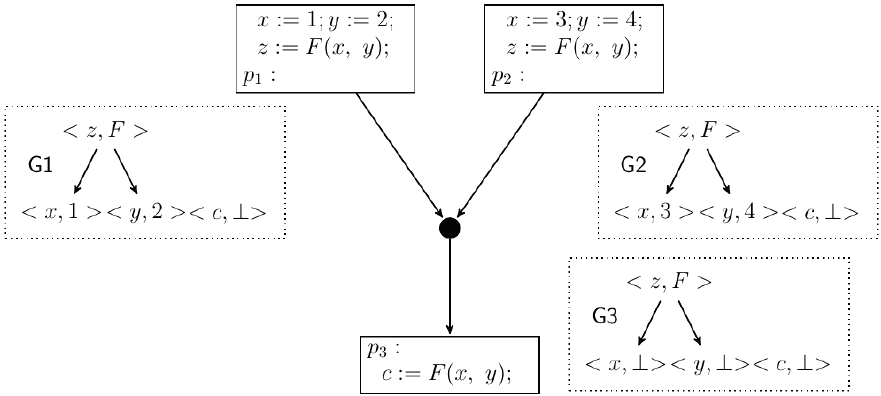Join of SEDs: Gi is the SED at program point pi. G3= Join(G1, G2).

## 3. GVN-GN and Detection of Value Based Redundancies

Let us now look at how we can make use of the equivalence information computed by GVN-GN in detecting value based redundancies. Consider a node n that contains an expression e. Let Gin be the SED computed by the algorithm at the entry of n. If eTerms (η) for some node η in Gin, then we can conclude that whenever control reaches the entry of n, an expression equivalent to e has been already computed and hence, the occurrence of e in n is redundant. For example in Fig. 2, for the SED G3, F(x, y) ∈ Terms (z, F). This indicates that when control reaches the point p3, in every path reaching p3, an expression equivalent to F(x, y) is computed. Hence, it is clear that the occurrence of F(x, y) in the last node is redundant.

What we have shown in Fig. 2 is an example for which the algorithm works fine. However, we have observed that there are cases for which the computed SED does not have the information required for redundancy detection, thereby limiting the use of this algorithm in related applications. In the following section, we show some examples of equivalences missed by GVN-GN.

### 3.1 Case of Missing Equivalences

Fig. 3 shows an example which, similar to Fig. 2, is a case where the occurrence of F(x, y) in the last node is redundant. We will now show that with the equivalence information computed by GVN-GN, it is not possible to detect this redundancy. The basic step in the Join algorithm invokes Intersect ( ) for each variable. For the variable a, Intersect (<a, F>, <a,>) results in <a,> in G3. For the variable b, Intersect (<b,>, <b, F>) results in <b,> in G3. The case for the variables x, y and c is also similar. In G1, F(x, y) ∈ Terms (<a, F>) and in G2, F(x, y) ∈ Terms (<b, F>). But for no node η in G3, F(x, y) ∈ Terms (η). As such, the redundancy in this case goes undetected. To have F(x, y) represented by a node in G3, there should be an invocation of Intersect (<a, F>, <b, F>). However, this is never invoked from Join (G1, G2).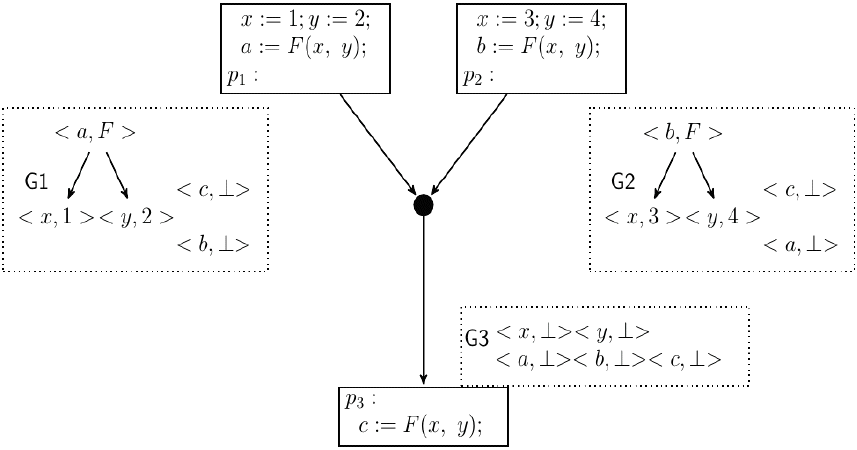Case of missing equivalences: for program point pi, Gi is the SED that GVN-GN computes. G3= Join(G1, G2). Intersect (<a, F>,<b, F) is not invoked.

The examples in Figs. 2 and 3 are similar except in the names of variables. The reason why Gulwani and Necula  fails in the second example is that there are no common variables labeling the nodes representing the expression F(x, y). This will be the case with any GVN algorithm that detects variable based equalities. The example clearly shows that this is not enough for redundancy detection in general.

Fig. 4 shows an additional example in which all the occurrences of F(x, y) are Herbrand equivalents. The node <c, F> in G1 represents the equivalence class [c, F(x, y), F(1, y), F(x, 2), F(1, 2)] and the node <d, F> in G2 represents the equivalence class [d, F(x, y), F(1, y), F(x, 2), F(1, 2)]. But there is no node in G3 that represents the equivalence class [F(x, y), F(1, y), F(x, 2), F(1, 2)]. Here, whenever control reaches p3, an expression equivalent to F(x, y) is already computed. However, there is no way to deduce this information from the SED G3.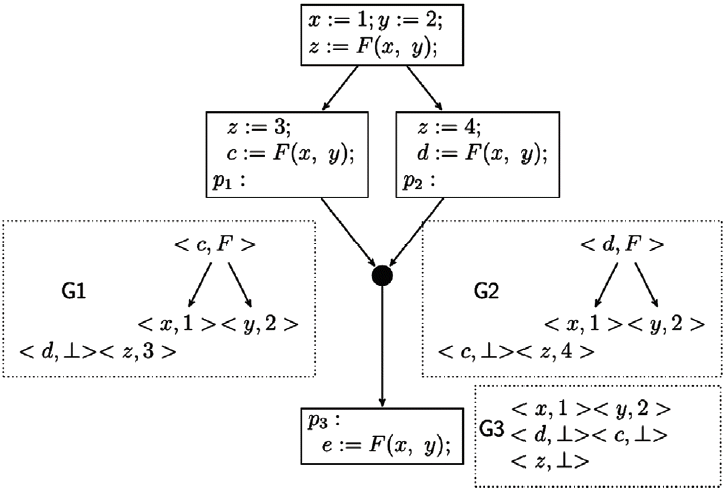Case of missing equivalences: for program point pi, Gi is the SED that GVN-GN computes. The expression F(x, y) and its equivalent expressions are not represented in the SED G3.

## 4. Anticipability-Based Join

The basic problem with the Join algorithm in GVN-GN is that it computes intersection of only those SED nodes having at least one common variable (see line 3 of the Join algorithm: for each variable x ∈ T . . . Intersect (NodeG1(x), NodeG2(x));). For the example in Fig. 3, in G1, F(x, y) ∈ Terms (<a, F>) and in G2, F(x, y) ∈ Terms (<b, F>). Now, to have F(x, y) represented by a node in G3, there should be an invocation of Intersect (<a, F>, <b, F>). In general, if there is an expression e such that e ∈ Terms (η1) and eTerms (η2) for some nodes η1 of G1 and η2 of G2, then to have e represented by a node in the resulting SED there should be an invocation of Intersect(η1, η2). This suggests that to have the required information at a join point the Join algorithm should compute the intersection of every pair of nodes of the two SEDs that have at least one common expression. Hence, a solution that will enable the algorithm to detect these kinds of equivalences is to modify the Join algorithm in such a way that it computes the intersection of every pair of nodes in the two SEDs

The approach of computing a pairwise intersection of the SED nodes will yield a precise algorithm. But theoretically, when we compute the join of n SEDs, it can result in an SED whose size is exponential in n. Furthermore, it can result in SED nodes representing non-program expressions. Here it is important to observe that for the purpose of redundancy detection, we do not need to keep all of the resulting nodes. The idea is to keep only those nodes η such that eTerms (η) for some expression e that is anticipable at the join point. This requires a preprocessing stage to compute the set of anticipable expressions at every program point.

Fig. 5 shows the SED computed by this approach for the example in Fig. 3. The intersection of <a, F> in G1 and <b, F> in G2 results in the node <ϕ, F> in G3, which represents the class [F(x, y)]. It should be noted that Gulwani and Necula  consider nodes like <ϕ, F> with an empty set of variables to be unnecessary. But in fact, these are necessary (as will be shown later) and as such, the proposed method will retain such nodes.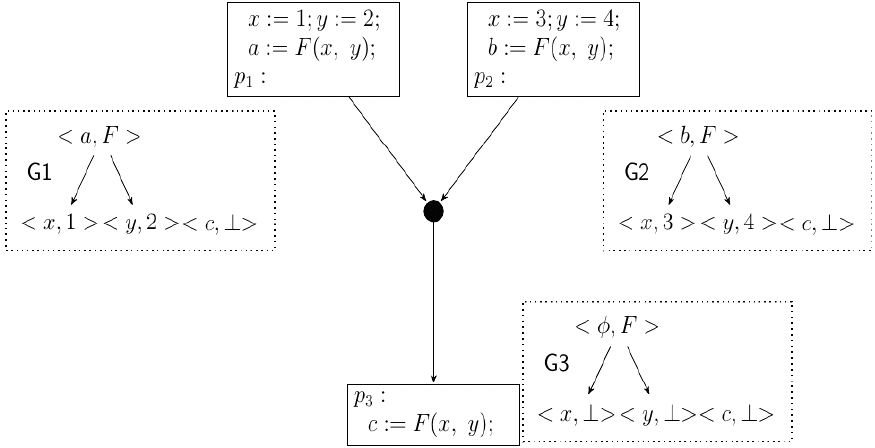Anticipability-based Join: G3 is the SED resulting from the join of G1 and G2. The expression F(x, y) is represented by <ϕ, F> in G3.

Fig. 6 shows the SED computed by the proposed method for the example in Fig. 4. The intersection of <c, F> in G1 and <d, F> in G2 results in the node <ϕ, F> in G3, which represents the class [F(x, y), F(x, 2), F(1, y), F(1, 2)]. Since F(x, y) is anticipable, the method retains this node.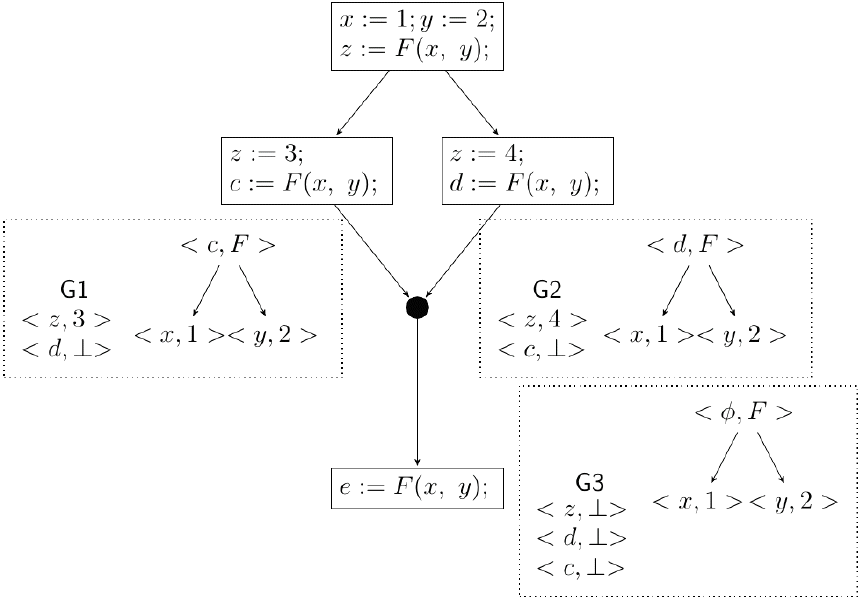Anticipabilty-based Join: G3 is the SED resulting from the join of G1 and G2.

### 4.1 Improved Algorithm

The following function Join_Improved shows the improved Join algorithm that computes the join of SEDs G1 and G2. The argument s′ is similar to that in GVN-GN. The additional argument ant_exp is the set of expressions that are anticipable at the join point. We assume that the set of anticipable expressions are precomputed at every program point using the classical anticipability analysis [9, 10]. T is the set of all program variables

Join-Improved (G1, G2, s′, ant_exp) =
1     for all nodes η1 ∈ G1 and η2 ∈ G2
2              memoize [η1, η2]:= undefined;
3     G = ϕ_;
4     for each variable x ∈ T in the order <G1 do
5              counter := s′;
6              Intersect (NodeG1(x), NodeG2(x));
7     for each expression e ∈ ant_exp do
8              counter := s′;
9              Intersect (FindNode (G1, e), FindNode (G2, e));
10     return G;


The function FindNode (G, e) is similar to GetNode (G, e) in GVN-GN, except that if there is no node in G representing e, then instead of extending G with a new node, the function returns <ϕ,>. The definition of FindNode (G, e) is given below.

FindNode (G, e) =
1      match e with
2 y: return NodeG(y);
3 F(e1, e2):let η1 = FindNode(G,e1)and η2 = FindNode(G,e2)
4             in if (<V, F (η1, η2)>) ∈ G return (<V, F (η1, η2)>);
5                                     else return (<ϕ, ⊥ >);


## 5. Correctness Proof and Analysis

In this section, we present the correctness proof and time complexity analysis of the improved algorithm.

### 5.1 A Note on the Correctness Proof in GVN-GN

The Soundness and Completeness proof of Join (Lemma 4) in  relies on the property of Intersect, which is stated as Proposition 3 in . With respect to the examples we discussed in the previous sections, we can see that there are cases where this property does not hold. We now state the property as given in  (Terms (ηi) denotes the set of terms represented by the node ηi).

#### Proposition 3 (as given in )

Let η1= <V1, t1> and η2 =<V2, t2> be any nodes in SEDs G1 and G2, respectively. Let η = <V, t> = Intersect (η1, η2). Suppose that η ≠ <ϕ, ⊥>; hence, the function Intersect (η1, η2) adds the node η to G. Let α be the value of the counter variable when Intersect (η1, η2) is first called. Then,

• (P1) Terms (η) ⊆ Terms (η1) ∩ Terms (η2).

• (P2) Terms (η) ⊇ {e |e ∈ Terms (η1), e ∈ Terms (η2), size (e)α}.

Now, for the example shown in Fig. 4, let η1 = <c, F>, in G1, and η2 = <d, F>, in G2. We get Intersect (η1, η2) = <ϕ, F><ϕ,>. But we have already seen that since the nodes have no common variables, the Join algorithm never invokes Intersect (η1, η2). Hence, against what is stated in the proposition, the node <ϕ, F> is not added to G3. The expression F(x, y) ∈ Terms (η1) and F(x, y) ∈ Terms (η2). But for no node ηi ∈ G3, F(x, y) ∈ Terms (ηi). Hence, the (P2) part of Proposition 3 does not hold in this case. We can see that with the Join algorithm in GVN-GN, Proposition 3 holds for the case of nodes η1= <V1, t1>, η2 = <V2, t2> such that V1V2ϕ. However, it need not hold if V1 ∩ V2 = f, except when Intersect (η1, η2) is invoked recursively from another Intersect call.

### 5. 2 The Completeness Proof of Join_Improved

The algorithm Join_Improved invokes Intersect (η1, η2) for a pair of nodes (η1, η2) from the two SEDs such that either V ∈ (Terms (η1) ∩ Terms (η2)) for some VT or e ∈ (Terms (η1) ∩ Terms (η2)) for some expression e that is anticipable at the join point. This can result in nodes of the form <ϕ, t> where t is either F or c, for some constant c. Now, since t ≠ ⊥, Line 11 of Intersect will add the resulting node to G3. Therefore, we restate Proposition 3 as follows:

#### Proposition 3′.

Let η1= <V1, t1> and η2 =<V2, t2> be any nodes in SEDs G1 and G2, respectively such that either V ∈ (Terms (η1) ∩ Terms (η2)) for some V T or e (Terms (η1) ∩ Terms (η2)) for some expression e that is anticipable at the join point. Let η = <V, t> = Intersect (η1, η2). Suppose that η ≠ <ϕ, ⊥>; hence, the function Intersect (η, η2) adds the node η to G. Let α be the value of the counter variable when Intersect (η1, η2) is first called. Then,

• (P1) Terms (η) ⊆ Terms (η1) ∩ Terms (η2).

• (P2) Terms (η) ⊇ {e |e ∈ Terms (η1), e ∈ Terms (η2), size (e)α}.

Now, we state the following Lemma on the Soundness and Completeness of Join_Improved (Terms (Gi) denotes the set of terms represented by the nodes of SED Gi):

#### Lemma 2′ (Soundness and Completeness of Join_Improved)

Let G = Join_Improved (G1, G2, s). Then,

1. If Ge1 = e2, then G1e1 = e2 and G2e1 = e2. If G1e1 = e2 and G2e1 = e2, such that size (e1)s and size (e2)s then Ge1 = e2

2. For an expression e of the form F (e1, e2), if e ∈ Terms (G), then e ∈ Terms (G1) and e ∈ Terms (G2). If e ∈ Terms (G1) and e ∈ Terms (G2), such that size (e)s, and e is anticipable at the join point, then e ∈ Terms (G).

As in , the proof of this Lemma follows from Proposition 3′ and the definition of ⊨.

### 5. 3 Fixed Point Computation

We have seen that the Join_Improved algorithm does intersections of even pairs of nodes with no common variables. We limit the number of nodes based on anticipability to at most ne, where ne is the number of expressions (including variables and constants) in the program. This implies that the improved algorithm needs to execute each flowchart node at most ne times (as in , we assume the standard work list implementation ).

### 5. 4 Correctness of the Algorithm

The Soundness Theorem and the Completeness Theorem for the improved algorithm are given below:

#### Theorem 2′ (Soundness Theorem)

Let G be the SED computed by the algorithm at some program point P after fixed point computation. Then,

1. if G ⊨ e1 = e2, then e1 = e2 holds at program point P.

2. if e ∈ Terms(G), then either e, or an expression equivalent to e, is available at P.

As stated in , the Soundness proof directly follows the soundness of the assignment operation, the non-det-assignment operation, and the soundness of Join_Improved.

#### Theorem 3′ (Completeness Theorem)

Let G be the SED computed by the algorithm at some program point P after fixed point computation.

1. if e1 = e2 is an equivalence that holds at P such that size(e1) ≤ s and size(e2) ≤ s, then Ge1 = e2.

2. if e is an expression that is available at P such that size(e) ≤ s and e is anticipable at P, then e ∈ Terms(G).

The proof is similar to that in , except that in order to prove the second part of the above theorem, we extended Lemma 5 of GVN-GN  to include the following statements: Suppose an expression equivalent to e (with the same restrictions on size (e)) is available at P along every path in S, such that e is anticipable at P, then, e ∈ Terms (G).

### 5. 5 Complexity Analysis

It is now clear that the improvements make the algorithm more precise. We now show that even with these improvements, the time complexity will still remain polynomial. With the additional step in Join_Improved, the number of nodes in an SED is at most ne, where ne is the number of expressions in the program. Therefore, a Join_Improved will invoke at most O(ne2) Intersect calls. The running time of Join_Improved (G1, G2, s′) will be O(s′×ne2), which is a polynomial in the number of expressions in the program (the use of the counter variable ensures that the depth of recursion is O(s′)). The size of the SED resulting after a Join_Improved call will be at most ne and thus, the time taken for computing the join of n SEDs will be O(n×s′×ne2).

## 6. GVN-GN: Problems with the Removal of SED Nodes

Here we will discuss an additional problem with GVN-GN that prevents the detection of some of the equivalences that even a local value numbering algorithm will detect. Fig. 7 shows a basic block in a program with the SEDs G1 and, G2 at program points p1 and p2, respectively. The expressions F(x, y) and the two occurrences of F(a, b) are equivalent and this equivalence will be detected by a local value numbering algorithm . It goes undetected in Gulwani and Necula  because of the reasons laid out below.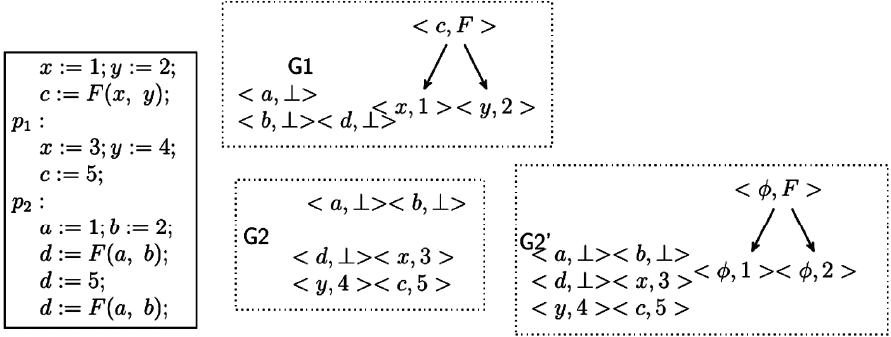Removal of “unnessary” nodes: G1 and G2 are the SEDs at points p1 and p2, respectively. G2 is the SED required at p2.

In Section 3.1 of Gulwani and Necula , it is stated that the transfer functions may yield SEDs with unnecessary nodes, and that these unnecessary nodes may be removed (a node is considered unnecessary when all of its ancestor nodes or all of its descendant nodes have an empty set of variables). Also, it is stated in Section 5.1 that the data structure (SED) represents only those partition classes that have at least one variable. G2 is the SED that would have been computed if there is no removal of SED nodes. Nevertheless, the algorithm considers the three nodes <ϕ, 1>, <ϕ, 2> and <ϕ, F> as unnecessary and hence these nodes will be removed. This results in SED G2.

### 6.1 Missing Equivalences

It can be observed that the node <ϕ, F> in G2 represents the expression F(1, 2), which is equivalent to F(x, y) and F(a, b). With the removal of this node, we lose the information that the expressions F(x, y) and F(a, b) are equivalent. Similarly, since the variable d is redefined after the first assignment d := F(a, b), the equivalence between the two occurrences of F(a, b) goes undetected.

#### 6.1.1 The solution

From the above example, it is clear that the problem is due to the removal of some necessary nodes, which the algorithm considers to be unnecessary. The simple solution is to retain all such nodes. In that case, for the above example, the SED reaching the input point of d:= F(a, b) will have a node representing the expression F(a, b), which will indicate that an expression equivalent to it has already been computed.

## 7. Conclusion

The main application of GVN is for code optimization in compilers, especially in detecting value based redundancies. The examples shown in Figs. 3 and 4 for the case of missing equivalences are instances of the classical common subexpressions. The example in Fig. 7 is an instance of local value numbering. These are enough evidence to show that the improvements are necessary for the practical adaptation of this GVN algorithm  in code optimizations. The suggested improvements result in a more precise and polynomial time algorithm for GVN.

## References

1. Gulwani S, Necula GC. A polynomial-time algorithm for global value numbering. Science of Computer Programming 64(1):97–114. 2007;
2. Rosen BK, Wegman MN, Zadeck FK. Global value numbers and redundant computations. In : Proceedings of the 15th ACM SIGPLAN-SIGACT Symposium on Principles of Programming Languages (POPL1988). San Diego, CA; 1988. p. 12–27.
3. Ruthing O, Knoop J, Steffen B. Detecting equalities of variables: combining efficiency with precision. Static Analysis Heidelberg: Springer; 1999. p. 232–247.
4. Kildall GA. A unified approach to global program optimization. In : Proceedings of the 1st Annual ACM SIGACT-SIGPLAN Symposium on Principles of Programming Languages (POPL1973). Boston, MA; 1973. p. 194–206.
5. Appel AW. Modern Compiler Implementation in Java 2nd edth ed. Cambridge: Cambridge University Press; 2002.
6. Muchnick SS. Advanced Compiler Design Implementation San Francisco, CA: Morgan Kaufmann Publishers; 1997.
7. Alpern B, Wegman MN, Zadeck FK. Detecting equality of variables in programs. In : Proceedings of the 15th ACM SIGPLAN-SIGACT Symposium on Principles of Programming Languages (POPL1988). San Diego, CA; 1988. p. 1–11.
8. Knoop J, Ruthing O, Steffen B. Lazy code motion. In : Proceedings of the ACM SIGPLAN Conference on Programming Language Design and Implementation (PLDI1992). San Francisco, CA; 1992. p. 224–234.
9. Morel E, Renvoise C. Global optimization by suppression of partial redundancies. Communications of the ACM 22(2):96–103. 1979;
10. Paleri VK, Srikant YN, Shankar P. Partial redundancy elimination: a simple, pragmatic, and provably correct algorithm. Science of Computer Programming 48(1):1–20. 2003;
11. Briggs P, Cooper KD, Simpson LT. Value numbering. Software-Practice and Experience 27(6):701–724. 1997;
12. VanDrunen T, Hosking AL. Value-based partial redundancy elimination. Compiler Construction Heidelberg: Springer; 2004. p. 167–184.
13. Gargi K. A sparse algorithm for predicated global value numbering. ACM SIGPLAN Notices 37(5):45–56. 2002;
14. Nie JT, Cheng X. An efficient ssa-based algorithm for complete global value numbering. Programming Languages and Systems Heidelberg: Springer; 2007. p. 319–334.
15. Cytron R, Ferrante J, Rosen BK, Wegman MN, Zadeck FK. Efficiently computing static single assignment form and the control dependence graph. ACM Transactions on Programming Languages and Systems 13(4):451–490. 1991;
16. Steffen B, Knoop J, Ruthing O. The value flow graph: a program representation for optimal program transformations. In : Proceedings of the 3rd European Symposium on Programming. Copenhagen, Denmark; 1990. p. 389–405.

## Biography

Nabizath SaleenaShe received Ms. Nabizath Saleena received her M.Tech Degree in Computer Science & Engineering from IIT Madras, India. She is working as an Assistant Professor in the Department of Computer Science and Engineering, NIT Calicut. Her research is in the area of Program Analysis.

Vineeth PaleriHe received Prof. Vineeth Paleri received his Ph.D. in Computer Science from Indian Institute of Sciences, Bangalore, India. He is working as a Professor in the Department of Computer Science and Engineering, NIT Calicut. His research interests include Programming Languages and Compilers.